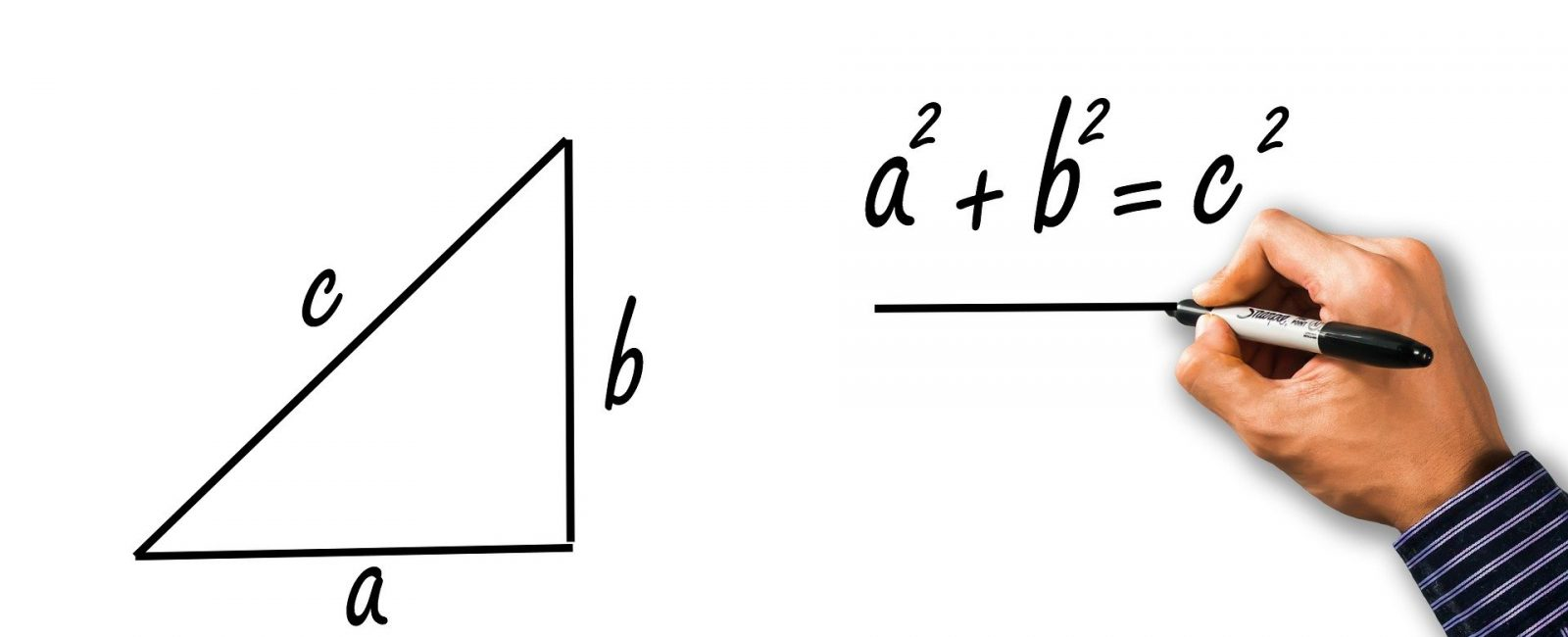# How to Find the Area and Perimeter of a Rectangle?

One of the geometric shapes which are very common is a rectangle. This shape is easy to find in everyday life and thus knowing the properties of rectangles is very helpful. While measuring a rectangle, there are two commonly needed dimensions one wants to know, the area while another is the perimeter of a rectangle.

Many of our land pieces and plots on which houses are constructed are often rectangular, and thus everyone needs to learn how to measure a rectangular shape.

Contents

### Area of a Rectangle

Let us assume we have a rectangular piece of land and we want to calculate its area. The area of a rectangle is required to be calculated when we want to know how much surface the rectangle can provide. For example, if one wants to grow some grains on rectangular farms, then the farm area will help us know how many seeds and manure would be required and how many grains we will be getting as the output. Or if we want to construct a house on a rectangular piece of land, then its area can help us know how much raw materials like cement, bricks, etc. would be required and its area would help us understand how big the rooms in the house can be. Or if one plans to make a swimming pool in a rectangular shape, then its area multiplied by the depth of a swimming pool would help us know how much water is required to be filled in the swimming pool.

### Calculating Area of Rectangle

Opposite sides of the rectangle are parallel to each other, and adjacent sides are perpendicular to each other. The area of rectangle is simply the multiplication of its two adjacent sides.

As we can see in the figure below, one side is often called ‘Length’, and the other side is known as ‘Breadth’. Thus, since the area of a rectangle is the multiplication of its adjacent sides. We can write that the area of the rectangle as below :

Area of rectangle = Length × Breadth

It is good to keep in mind that a rectangle area is always represented in square units.

For example, we have a rectangle with length = 10 meters, and breadth = 5 meters then the area of this rectangle using our formula above would be 10 meters * 5 meters = 50-meter square.

Likewise, if length of rectangle = 10 centimeters and breadth of rectangle = 3 centimeters

Then, the area of the rectangle would be; 10 centimeters * 3 centimeters = 30-centimeter square

## Perimeter of Rectangle

If we have a farmland piece and it is rectangular on which there is a requirement to erect fencing of barbed wires. Now, how much fencing would be required? In this scenario, merely finding out the rectangular farmland area would not be enough as we need to know the length of its sides to know how much fencing would be required. This is one of the examples of the utility of the perimeter of the rectangle. The perimeter of a rectangle or in fact perimeter of any shape is the total length of all its sides. For example, in a circle, the perimeter is called circumference is the total length of the circle. In the case of a rectangle perimeter of the rectangle would similarly be the total length of its sides.

## Calculating Perimeter of a Rectangle

We have seen above that rectangle has four sides; opposite sides are parallel and equal in length. So we have to add the rectangle’s size to the breadth of the rectangle, and we need to do it two times as there are two long sides and two breadth sides.

So, the perimeter of the rectangle would be 2 × (Length + breadth)

For more interesting applications of rectangles in real life and how their area and perimeter problem solving occurs one can visit Cuemath to find a suitable math tutor or easy to download worksheets are also available to have a good practice on the problems pertaining to rectangles.